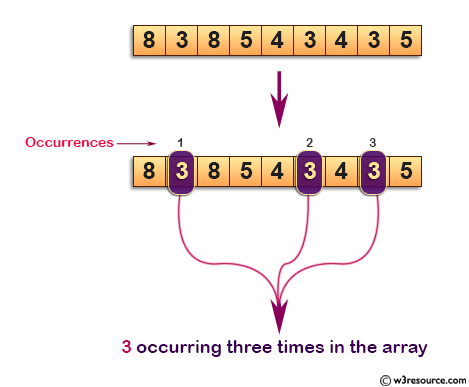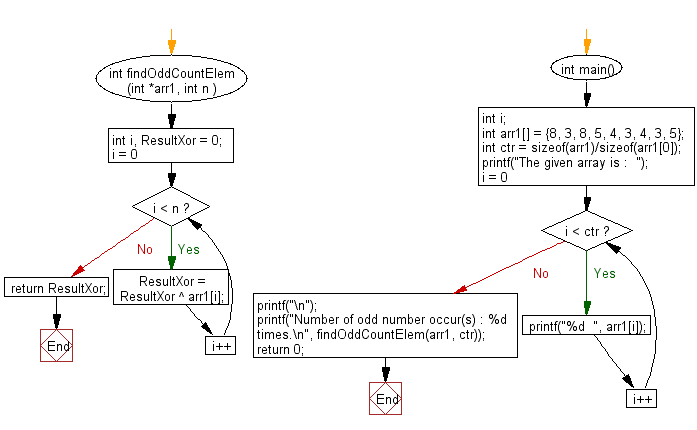﻿ C : Number occurring odd number of times in an array

# C Exercises: Find the number occurring odd number of times in an array

## C Array: Exercise-34 with Solution

Write a program in C to find the number occurring odd number of times in an array.
All numbers occur even number of times except one number which occurs odd number of times.

Pictorial Presentation:Sample Solution:

C Code:

``````#include <stdio.h>

int findOddCountElem (int *arr1, int n )
{
int i, ResultXor = 0;
for(i = 0; i < n; i++)
{
ResultXor = ResultXor ^ arr1[i];
}
return ResultXor;
}

int main()
{
int i;
int arr1[] = {8, 3, 8, 5, 4, 3, 4, 3, 5};

int ctr = sizeof(arr1)/sizeof(arr1);
printf("The given array is :  ");

for(i = 0; i < ctr; i++)
{
printf("%d  ", arr1[i]);
}
printf("\n");

printf("Number of odd number occur(s) : %d times.\n", findOddCountElem(arr1, ctr));
return 0;
}
```
```

Sample Output:

```The given array is :  8  3  8  5  4  3  4  3  5
Number of odd number occur(s) : 3 times.
```

Flowchart :C Programming Code Editor:

Improve this sample solution and post your code through Disqus.

What is the difficulty level of this exercise?

Test your Programming skills with w3resource's quiz.

﻿

## C Programming: Tips of the Day

Returning an array using C

You can't return arrays from functions in C. You also can't (shouldn't) do this:

```char *returnArray(char array []){
char returned ;
//methods to pull values from array, interpret them, and then create new array
return &(returned); //is this correct?
}
```

returned is created with automatic storage duration and references to it will become invalid once it leaves its declaring scope, i.e., when the function returns.

You will need to dynamically allocate the memory inside of the function or fill a preallocated buffer provided by the caller.

Dynamically allocate the memory inside of the function (caller responsible for deallocating ret)

```char *foo(int count) {
char *ret = malloc(count);
if(!ret)
return NULL;

for(int i = 0; i < count; ++i)
ret[i] = i;

return ret;
}
```

Call it like so:

```int main() {
char *p = foo(10);
if(p) {
// do stuff with p
free(p);
}

return 0;
}
```

Ref : https://bit.ly/3yFIeao

We are closing our Disqus commenting system for some maintenanace issues. You may write to us at reach[at]yahoo[dot]com or visit us at Facebook